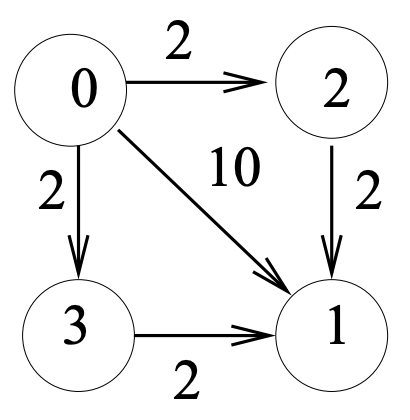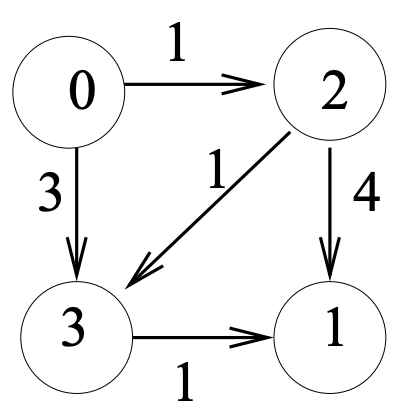시간 제한 메모리 제한 제출 정답 맞은 사람 정답 비율
2 초 512 MB 0 0 0 0.000%

## 문제

A graph is made up of a set of nodes and a set of links. A link connects two nodes. For example, Figure 1 shows a simple graph with 4 nodes and 5 links. In the figure, each link has a specific direction, going from the originating node to the destination node. Each link has a cost attached to it. The m nodes of a graph are identified by the integers 0, 1, ..., m − 1.

A path connects one node to another, following the direction of links from node to node. The length of a path is the number of links used. The cost of a path is the sum of link cost over the entire path. For a given graph, your task is to find the minimum cost among the costs of all the shortest paths between Node 0 and Node 1. A shortest path with the minimum cost is called a minimum-cost shortest path.Figure 1 Figure 2

For example, again consider Figure 1. The shortest path from Node 0 to Node 1 is the 1-link path 0 → 1 with a path cost of 10. While there are cheaper paths: 0 → 2 → 1 and 0 → 3 → 1, they are longer (2 links). Therefore, 0 → 1 is the minimum-cost shortest path.

Consider another example, Figure 2. There are two shortest paths of length 2, the path 0 → 3 → 1 has a lower cost (cost = 4) than the path 0 → 2 → 1 (cost = 5). Another path 0 → 2 → 3 → 1 (cost = 3) is cheaper but longer. Therefore, the minimum-cost shortest path is 0 → 3 → 1.

## 입력

The first line contains two integers m and n separated by a space, where m is the number of nodes and n is the number of links. The links of the graph and their costs are given by the next n lines. Each line has three integers (with a space between two adjacent integers), denoting Source-Node Destination-Node Link-Cost.

There are at most 100 nodes, and nodes are numbered from 0 to 99. There are at most 1000 links and link cost varies between 0 and 215 − 1.

## 출력

The output file contains a single integer which is the path cost of a minimum-cost shortest path from Node 0 to Node 1.

Note that while there may be multiple minimum-cost shortest paths, the cost of these paths are the same.

## 예제 입력 1

4 5
0 2 2
0 3 2
0 1 10
2 1 2
3 1 2


## 예제 출력 1

10